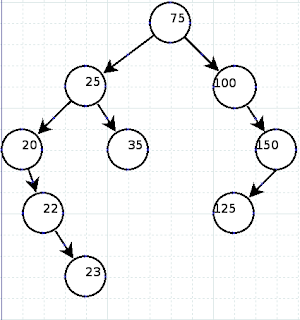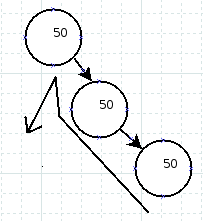## Tuesday, September 9, 2008

### Binary Trees - Does it matter?

Ever programmed a Binary Tree before? If you took computer science classes past an introductory level, you probably studied the theory before. But to what extent do decisions matter?

To review for those of you who don't quite recall what a binary tree is. You have a series of nodes, each node contains data, some or all of which is the key, up to two pointers to children nodes, and possibly other meta data. The most popular type of binary tree is the Binary Search Tree, where each node conceptually has nodes with lesser keys descending on the left, and nodes with greater keys descending on the right.How to implement a left and right can be done with two node pointers called left and right, lesser and greater, an array of two node pointers, or any other method which makes sense to you.

All operations begin with the topmost node in the figure above, known as the root, head, or top node of the tree. To find a node with a particular key, one compares it against the current node, if it found it good, otherwise, if it's less, take the left route, otherwise the right. If there is no path left or right as requested, since there is only a null pointer there, then a node with a matching key is not in the tree. At each node reached, the same algorithm is applied. In this instance, a Binary Tree is very similar to Binary Search, each check effectively cuts the data in half, leading to very efficient searches.

Adding data to a tree is very much the same. The data is encapsulated in a node, which again goes down the tree finding where the data should belong, in this case, once a null pointer is encountered while trying to traverse the tree further, it is replaced by a pointer to the new node.

Deleting data can be a bit trickier. If a node has no children, deleting a node is quite straight forward. If the node only has a path left or right, one simply cuts out the node being deleted, and makes the pointer once pointing to it now point to the child. However, for removing a node which has two children descending from it, there's a number of methods that can be applied.

The simplest method is to make one child a replacement, and add the other child onto the child replacing, meaning add the left node to the right or the right to the left.

Look what happens when deleting the root node, which in this case has two children.The node with a key of 50 is deleted which had two children, 25 and 100. Either 25 becomes the new root, with 100 as the rightmost of 25's descendants, or 100 becomes the new root, with 25 as the leftmost of 100's descendants.

The only problem with this algorithm is that it can quickly make the tree become unbalanced, degrading into a linked list. A tree should be as close to balanced as possible, so search or addition can be done with cutting half the tree out per each node looked at. An unbalanced tree would make each operation generally consist of looking at most of the nodes in the tree, which is inefficient.

Therefore, the generally proposed algorithm is to find the next or previous node by key, and move it to replace the node being deleted. First lest us understand tree order.

A binary tree can be processed in direct order using the following algorithm.
`function process_node(Node *node){  if (node->has_left()) { process_node(node->left()); }  process_node_data(node->data());  if (node->has_right()) { process_node(node->right()); }}`

Which is plain English means handle left, then current, then right, in a recursive fashion. Because a Binary Tree can be processed in this fashion, in addition to being a nice data structure, it can also be used as the basis of a sorting algorithm. All data is encapsulated in a node and is added to the tree, then it is traversed in order as just described, placing the data back into the original data structure, and then the tree is destroyed.

Now understanding this, the node prior to any particular node in regards to its key is always one left, and then rightmost, and the node after a particular key is one right, and then left most.

And the delete:50 is deleted, and the previous or next one takes its place. In this case, 35 being the previous, and 75 being the next one.

Now to get to the meat of the article, does it matter? Does what matter?

First off, we didn't at all mention how to handle nodes which have equivalent keys. Back when I was in college learning computer science, we were told to just throw it down the right or the left, but be consistent about it. Also, when it comes to deleting, take the previous or the next, but again, it doesn't matter, as long as one was consistent about it. Or perhaps in this latter case, alternate sides per delete.

But the question we must ask is: "Does it matter?"

And the answer which they at the very least didn't teach me when I was in school, yes, it does matter!

When one sorts, one can do a stable or an unstable sort. Stable means that if two elements had equivalent keys, when sorting, the order of those nodes would be preserved. Meaning if we had: "50 C, 45 A, 23 C, 50 B, 75 D, 50 A", and the numbers would make up the key, but not the letters, then it would sort to: "23 C, 45 A, 50 C, 50 B, 50 A, 75 D", keeping the order of C,B,A among the elements with a key of 50. Unstable would mean that the relative order of those 50s is not guaranteed to be kept when sorting.

A binary tree can only be used as a stable sort if equal data is always placed on the right side. This is because the order of succession is left, current, right, and if it was placed on the left, it would mean the node added later came before the current node.

What not to do:However, just putting equal data on the right isn't the end of the matter yet. Most good Binary Trees, such as a Red & Black Tree will implement some kind of self balancing system, so areas which are starting to become linked lists rotate to become balanced trees.